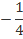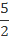Alternatively, you can register/login faster using

Register Free!
 QUESTION OF THE DAY
Question of the day
HOME > MBA PREP CENTRE > DAILY TEST PREP > QUESTION OF THE DAYSee More Questions
Question of the Day (10-Oct-20)

 Views : 4093 Rated 4.0 by 10 Users
Quadratic functions f(x) = −x2 + ax + b and g(x) = x2 + cx + d are defined for real values of x such that f(x) < 0 for all values of x, except x = 2 and g(x) > 0 for all values of x except 2 ≤ x ≤ 3. If h(x) = max [f(x), g(x)] then for what value of x is the value of the function h(x) minimum?
 OPTIONS 1) 2 2) 0 3)4)5) None of these